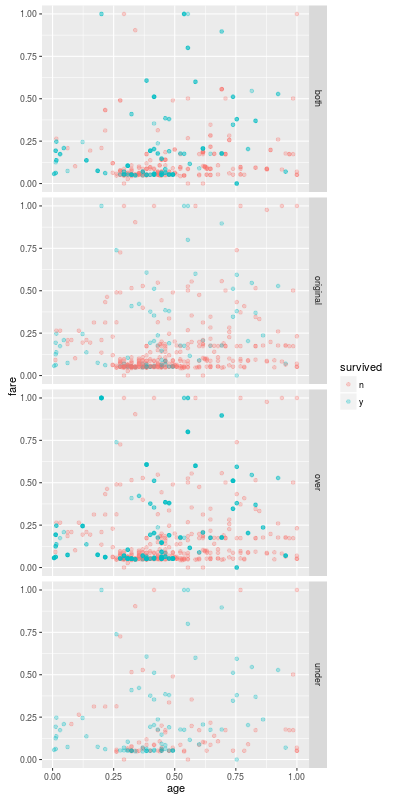Data Experiment #07-2 Imbalanced classification problems

In the last entry we looked at the imbalanced classification problems. In this entry we try sampling methods and observe what happens.

NB: We show only part of code and the result. The complete code can be found my BitBucket repository.

## Data

We use the famous Titanic data. If you have tried a famous competitoin, you must know that most of men died. That is the "survived" variable is skewed after restricting the data to male samples. We can find the data in PASWR package.

library(caret); library(ggplot2); library(plyr); library(dplyr); library(tsne)
library(ROSE) ## library for sampling methods

df <- PASWR::titanic3 %>%
filter(sex=='male') %>%
select(-name,-sex,-ticket,-boat,-body,-home.dest) %>%
mutate(cabin=ifelse(cabin=='',1,0),
survived=ifelse(survived==1,'y','n'))


For simplicity we use the complete rows (i.e. ignore samples containing NA, etc.). We split the data into a training set and a test set:

set.seed(1)
seed=4)$data  The oversampled training set contains 314 negative samples and 314 positive samples. Since the actual number of positive samples is 81, each positive sample appears 3.87 times in df.os on average.  method acc.train acc.test y.rate recall precision F1.score 1 nobody 0.7949367 0.7938931 0.0000000 0.0000000 0.0000000 0.0000000 2 glmnet 0.7113924 0.7099237 0.3511450 0.6481481 0.3804348 0.4794521 3 svmRadial 0.7772152 0.7404580 0.2900763 0.5740741 0.4078947 0.4769231 4 rf 0.9848101 0.7519084 0.2328244 0.4629630 0.4098361 0.4347826 5 xgbTree 0.9518987 0.7442748 0.2480916 0.4814815 0.4000000 0.4369748  In comparison with undersampling, the predicted rate of "y" is smaller, but it is still larger than the actual positive rate (i.e. around 0.2). The recall is slightly smaller but the precision is improved so that there is no remarkable difference of F1-scores. We should note that the size of the training set becomes relatively large after the oversampling. While the original training set consists of 395 samples, df.os consists of 628 samples. ### Applying both undersampling and oversampling df.both <- ovun.sample(survived~.,data=df.train,method='both',N=400,seed=5)$data


The data frame df.both contains 214 negative samples and 186 positive samples.

     method acc.train  acc.test    y.rate    recall precision  F1.score
1    nobody 0.7949367 0.7938931 0.0000000 0.0000000 0.0000000 0.0000000
2    glmnet 0.7468354 0.7175573 0.3206107 0.5925926 0.3809524 0.4637681
3 svmRadial 0.7696203 0.6946565 0.3282443 0.5555556 0.3488372 0.4285714
4        rf 0.8607595 0.6908397 0.3549618 0.6111111 0.3548387 0.4489796
5   xgbTree 0.8481013 0.6984733 0.3167939 0.5370370 0.3493976 0.4233577


The result can depend on the number of samples N and the proportion p of the positive class. (The default is p=0.5.) But our result is similar to the result of the undersampling.## Next Entry

The undersamplings and oversamplings are both very easy to understand. In the next entry we try slightly complicated method for a imbalanced classification model.

### Tips

• You might improve either recall or precision by adjusting the size of the sampled data. (That is the N option in the ovun.sample().)
• As we mentioned in the first entry, we rely on the caret package for turning meta parameter. That is because the goal of an imbalanced classification problem is not necessarily the high accuracy. So the choice of meta parameters depends on the question. Moreover the aim of this entry is an observation of the sample methods, not the careful turning for the data set. But we have to choose meta parameters, so we let caret choose them.
Categories: #data-mining